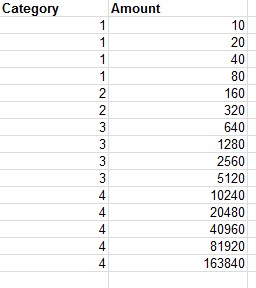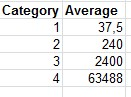# New to QlikView

Discussion board where members can get started with QlikView.

Announcements
Modernize Your QlikView Deployment webinar, Nov. 3rd. REGISTER
cancel
Showing results for
Did you mean:
HighlightedNot applicable

## Get data from existing chart tables

@

I have a very simple chart object and I want to create a new text box that shows a specific value from the chart (eg. the cell A1) multiplied by a standard Variable. How can I read-access the data from this cell of the chart in order to create an expression to the text box??

More specifically, I have loaded 5 dimension from a simple excel file. I have created a very simple chart pivot object on which i have selected on the dimension tab one dimension and on the expression tab i have selected an average function on another dimension from the total 5. The pivot chart object works fine. Now i want to calculate in text box an amount that makes use of a cell from the pivot chart object. How can i reach the value of this chart pivot object?? for example i want to calculate on the text box object the value of the cell [A,2] multiplied by a variable. Is there an function to get specific data from the pivot chart object making use of the object id for example??????

Message was edited by: Theofanis Hatzis

1 Solution

Accepted Solutions
HighlightedPartner

Average({\$<Category={1}>}Amount)*Variable1

+

Average({\$<Category={2}>}Amount)*Variable2

+

Average({\$<Category={3}>}Amount)*Variable3

+

Average({\$<Category={4}>}Amount)*Variable4

Any reason why you can't do it like this?

10 Replies
HighlightedCreator III

Is this something you would could use set analysis for?   Then you could take the set analysis result and multiply it by the variable?  Could you add some example?

HighlightedNot applicable

dear josh i edited my first post so you can review.

HighlightedCreator III

A2 is an expression that equals a value.  So the expression is something like:

AVG(Expression)

If you put this save value in a text object, and select 1 dimension, they should equal, then you can multiply this by a variable.  If you have to get the actual coordinates from the pivot table, I've never seen this situation.  Maybe someone else has and could help.  Good luck!!

HighlightedNot applicable

Yes I want exactly this. I want to get the data from actual coordinates from the pivot table(eg. A2) and use them on a calculation in a text box. I hope someone else knows about! Thank you very much mr Josh.

HighlightedPartner

As far as I know there is no way to select a single coordinate like you say, but I suppose there should be some other way.

How are you going to specify the coordinates? Are they fixed or are they coming from somewhere on the fly?

HighlightedNot applicable

Yes they are fixed. The pivot table chart object is 4x2 and I want to get the four cells of the second column. Is there any way to do it making use of the function : str GetObjectField ([index [, objectid]])  ?????

It returns string but I want the number not the string and also I dont know how to use the index parameter in order to specify the cell [B,1] for example.

I tried the following :

=GetObjectField(0,'CH02')

and it returned me the name of the first column.

HighlightedPartner

Why cant you just use set analysis to select dimensions in the text box?

What is changing in the pivot table that would change the output in the wanted columns?

HighlightedNot applicable

The excel table I have loaded the original data is the following:The pivot table chart object i have created (CH02) is the following:and I want to produce one number making use of the above averages. For example:

37,5 * Variable1 +240 *Variable2 + 2400* Variable3 + 63488*Variable4

HighlightedPartner

Average({\$<Category={1}>}Amount)*Variable1

+

Average({\$<Category={2}>}Amount)*Variable2

+

Average({\$<Category={3}>}Amount)*Variable3

+

Average({\$<Category={4}>}Amount)*Variable4

Any reason why you can't do it like this?